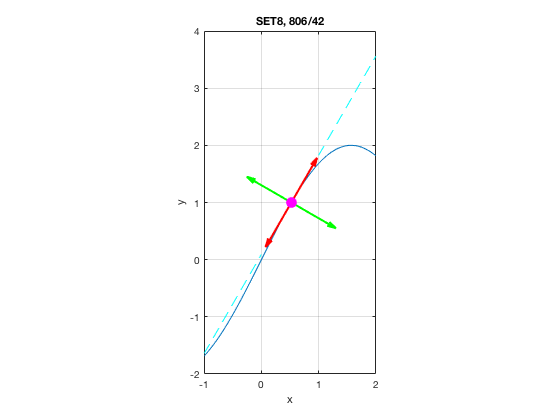# MATH 251: Calculus 3, SET8

## 12: Vectors and the Geometry of Space

### 12.2: Vectors

These problems are done with the CAS. See Hand Solutions for details.

#### 1. [805/8]

The figure provides the following infomation. By hand, we write w =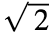i or w =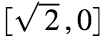.
s= sym(1/sqrt(2)), u = [-s s], v = [-s -s]
s =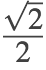u =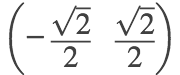v =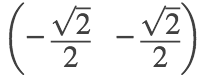w = -u - v
w =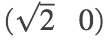figure
quiver(0,0, -s,s, 'LineWidth', 2); grid on; hold on
quiver(0,0, -s,-s, 'LineWidth', 2)
quiver(0,0, 2*s,0, 'LineWidth', 2)
axis equal; axis([-1 2 -1 1])
xticks(-1:2); yticks(-1:1)
xlabel('x'); ylabel('y')
title('SET8, 805/8')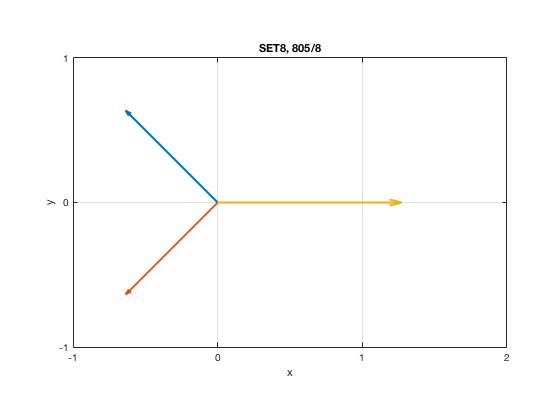#### 2. [805/14]

A = sym([0 6 -1]); B = sym([3 4 4]); AB = B - A
AB =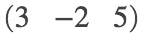figure
quiver3(0,6,-1, 3,-2,5, 'LineWidth', 4); grid on; hold on
quiver3(0,0,0, 3,-2,5, 'LineWidth', 4)
view(-60,30)
axis equal; axis([0 3 -2 6 -1 5])
xlabel('x'); ylabel('y'); zlabel('z')
title('SET8, 805/14')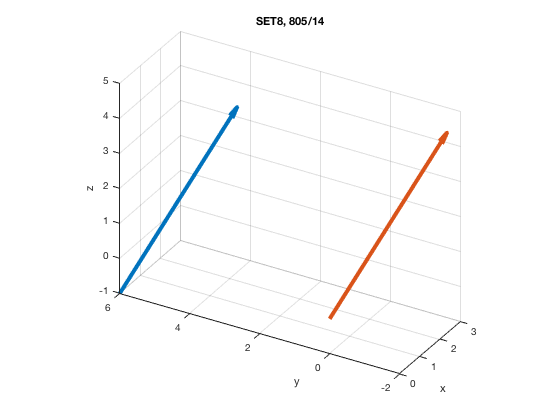#### 3. [805/22]

a = sym([8 1 -4]); b = sym([5 -2 1]);
a_plus_b = a + b
a_plus_b =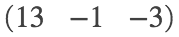linear_combo = 4*a + 2*b
linear_combo =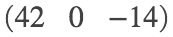length_of_a = norm(a)
length_of_a = 9
length_of_a_minus_b = norm(a-b)
length_of_a_minus_b =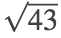#### 4. [805/26]

The vector w has length 4 and points in the same direction as v.
v = sym([6 2 -3])
v =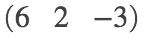length_of_v = norm(v)
length_of_v = 7
v_hat = v / length_of_v
v_hat =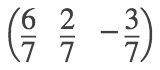w = 4*v_hat
w =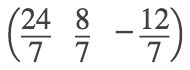length_of_w = norm(w)
length_of_w = 4

#### 5. [805/28]

The angle between the vector v = 8i+6j and the positive x-axis is obtained via slope (rise/run); roughly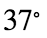.
atan2d(6,8)
ans = 36.8699

#### 6. [805/30]

The horizontal and vertical forces (in N) exerted on a sled are computed.
hv = 50*[cosd(38), sind(38)]
hv = 1×2
39.4005 30.7831

#### 7. [806/32]

The magnitude and angle (w.r.t. positive x-axis) of a resultant force is computed.
F1 = 20*[cosd(45), sind(45)]
F1 = 1×2
14.1421 14.1421
F2 = 16*[cosd(-30), sind(-30)]
F2 = 1×2
13.8564 -8.0000
F = F1 + F2 % rectangular components
F = 1×2
27.9985 6.1421
mag_F = norm(F) % in lb
mag_F = 28.6643
ang_f = atan2d(F(2),F(1)) % in degrees
ang_f = 12.3732

#### 8. [806/38]

The weight (in N) of a suspended chain is computed.
weight = 2*(25*sind(37))
weight = 30.0908

#### 9. [806/40]

Compute the resultant force F of the 3 constituent forces. Its negation G = -F is the counterbalancing force whose magnitude is computed.
F1 = 25*[1 0 0]
F1 = 1×3
25 0 0
F2 = 12*[cosd(100) sind(100) 0]
F2 = 1×3
-2.0838 11.8177 0
F3 = 4*[0 0 1]
F3 = 1×3
0 0 4
F = F1 + F2 + F3
F = 1×3
22.9162 11.8177 4.0000
G = -F
G = 1×3
-22.9162 -11.8177 -4.0000
mag_G = norm(G) % in N
mag_G = 26.0924

#### 10. [806/42]

The tangent line to the curve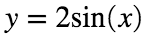atis computed.
1. The two unit tangent vectors parallel to the tangent line are found.
2. The two unit normal vectors perpendicular to the tangent line are found.
3. An illustrative figure is provided.
syms x y
f(x) = 2*sin(x)
f(x) =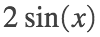Df(x) = diff(f(x), x)
Df(x) =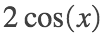m = Df(pi/6)
m =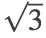L(x) = expand(f(pi/6) + m*(x - pi/6))
L(x) =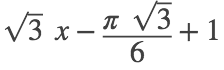P = [pi/6, f(pi/6)]
P =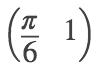v = [1, m]
v =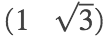T = v / norm(v)
T =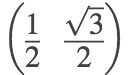N = [-T(2) T(1)]
N =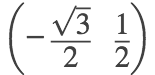%
figure
fplot(f(x), [-1 2], 'LineWidth', 1); grid on; hold on
fplot(L(x), [1 2], 'c--');
fplot(L(x), [-1 0], 'c--');
quiver(pi/6,1, 1/2, sqrt(3)/2, 'r', 'LineWidth', 2, 'MaxHeadSize', 0.6)
quiver(pi/6,1, -1/2, -sqrt(3)/2, 'r', 'LineWidth', 2, 'MaxHeadSize', 0.6)
quiver(pi/6,1, -sqrt(3)/2, 1/2, 'g', 'LineWidth', 2, 'MaxHeadSize', 0.6)
quiver(pi/6,1, sqrt(3)/2, -1/2, 'g', 'LineWidth', 2, 'MaxHeadSize', 0.6)
plot(pi/6, 1, 'mo', 'MarkerFaceColor', 'm', 'MarkerSize', 10)
axis equal; axis([-1 2 -2 4])
xlabel('x'); ylabel('y')
title('SET8, 806/42')